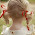# geeky.blogger

## Cyclic Coordinate Descent (CCD)

Tuesday, April 29, 2008 Posted by Ismail Habib
As I mentioned on previous post. This post will describe more about CCD in relation to Inverse Kinematics and Human Body Animation.

The basic idea of Cyclic Coordinate Descent (CCD) Method is performing iterative heuristic search for each joint angle, so at the end, the end-effector could reach a desired location in space. By doing iterative heuristic search, we try to find the best location of an articulated structure given its joint angles. This would result in the best (closest) distance of the end-effectors and the desired end points. The calculation is done one joint at a time, and in backward fashion, until we reached the parent joint. For calculating the joint angle, we also give certain limitation for the angle, just like the angle of a human. We implement this, so the animation wouldn’t do any strange movement. The first thing to do in CCD method is to determine the desired location of the end-effector (X). After that, we try to minimize the distance (ΔX) between end-effector position (X^) and the desired destination (X) as low as possible. We do this by increasing/decreasing the current joint angle of the end-effector. When the distance of the end-effector is as low as possible, it means that the current joint angle (Θ) has reached its maximum point, given the current position. Then we move backwards to the upper joint, where we then try again to minimize the distance between end-effector and destination point, but this time only altering the current upper joint. We do this until we reached the parent joint. An example can be seen in the following figure.Cyclic Coordinate Descent Method for: a) Rotational Joint and b) Prismatic Joint

For example, we can imagine when we try to move the whole hand. The first thing that we do is to calculate the minimum distance that could be reached by our hand only using the wrist joint. After that, we then move backwards and try manipulate the elbow joint and again calculate the minimum distance that could be reached by our hand. We do this until the last joint is manipulated, for instance, the shoulder joint, or even our backbone joint. Each human body joint are capable to rotate in one to three different axis (3-Dimension). This ability to rotate is called Degree of Freedom of a joint. So each joint could have 1 to 3 Degree of Freedom (DOF). Using CCD method, we try to find the best joint angle for x-axis, y-axis and z-axis for a joint given the coordinate of the destination point (i.e. the best joint angle of each axis that could make the end-effector closer to the destination point). Suppose a joint cannot move in an axis, the best joint angle for that axis is zero. After calculating both three joint angles for each axis, we then calculate the rotation matrix for each axis, using the following rotation matrix:Where RotX is the rotation matrix for x-axis, RotY is for y-axis and RotZ is for z-axis. We then combine these rotation matrixes into a single rotation matrix:

R = RotZ*RotY*RotX

Where R matrix is the rotation matrix of a joint. Applying rotation matrix of Joint(i) towards the end-effector will cause all the part from Joint(i) up to the end-effector to rotate. After that, the calculation will move backwards to Joint(i-1) and again we calculate the joint angle for Joint(i-1) and calculate the rotation matrix. In order to move end-effector given the rotation of Joint(i-1), besides rotation matrix of the current joint, we also need rotation matrix of the previous joint and the translation matrix of the previous link. The following is the translation matrix:Where dX, dY, dZ is the length of the link (i.e. the distance between two joints). For transforming the end-effector given the rotation of Joint(i-1), we need the matrix of R(i-1)*T(i)*R(i), where T(i) is the translation matrix from the current joint to the previous joint (i.e. length of the link) and R(i) is the rotation matrix from the previous joint. Keep in mind that the calculation is done backwards starting from the end-effector towards the parent joint.This calculation will continue until we reached the parent joint where there will be no more joints that could rotate after this joint. After reaching the parent joint, the calculated distance between current end-effector location and the desired location should be as minimum as possible.

### Related post

1.What about doing straight lines to the goal. Most of which will of course overs-shoot.
And decrease amount of joints each time. Until You reach first one.
You get bunch of errors ...

Has there been something which explored such idea?

Regards

hribek

2.Anonymous6:07 PM

there is a mistake at the RotZ matrix.
m33 needs to be a 1 instead a 0 !

3.Great post! It's a shame that I do not understand those formulas and equations.

4.5.6.7.8.9.10.11.12.13.14.15.16.17.18.19.20.21.22.23.24.25.26.27.28.29.30.31.32.33.34.35.36.37.شركة تنظيف بمكة شركة تنظيف بمكة
شركة تنظيف بالمدينة المنورة شركة تنظيف بالمدينة المنورة
شركة تنظيف بالجبيل شركة تنظيف بالجبيل
شركة تنظيف بالدمام شركة تنظيف بالدمام
شركة تنظيف بالخبر شركة تنظيف بالخبر

38.I admire the person who wrote this post, you are so talented, hope you will promote them and become more successful. I do not feel sorry for taking the time to read this post, it is really nice and useful to me, thanks for posting it.
jogos io gratis, cá koi mini, friv at school 2019, abcya 3